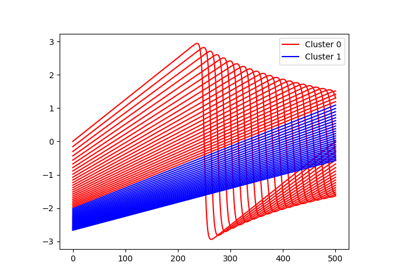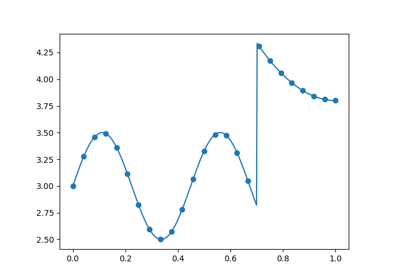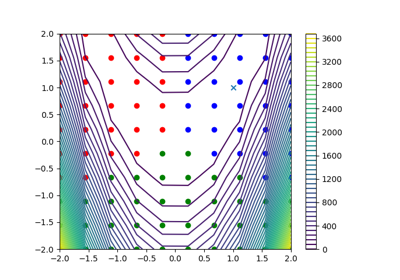gemseo / mlearning / regression

# moe module¶

Mixture of experts for regression.

The mixture of experts (MoE) model expresses an output variable as the weighted sum of the outputs of local regression models, whose weights depend on the input data.

During the learning stage, the input space is divided into $$K$$ clusters by a clustering model, then a classification model is built to map the input space to the cluster space, and finally a regression model $$f_k$$ is built for the $$k$$-th cluster.

The classification may be either hard, in which case only one of the weights is equal to one, and the rest equal to zero:

$y = \sum_{k=1}^K I_{C_k}(x) f_k(x),$

or soft, in which case the weights express the probabilities of belonging to each cluster:

$y = \sum_{k=1}^K \mathbb{P}(x \in C_k) f_k(x),$

where $$x$$ is the input data, $$y$$ is the output data, $$K$$ is the number of clusters, $$(C_k)_{k=1,\cdots,K}$$ are the input spaces associated to the clusters, $$I_{C_k}(x)$$ is the indicator of class $$k$$, $$\mathbb{P}(x \in C_k)$$ is the probability that $$x$$ belongs to cluster $$k$$ and $$f_k(x)$$ is the local regression model on cluster $$k$$.

class gemseo.mlearning.regression.moe.MOERegressor(data, transformer=None, input_names=None, output_names=None, hard=True)[source]

Mixture of experts regression.

Parameters:
• data (Dataset) – The learning dataset.

• transformer (Mapping[str, TransformerType] | None) – The strategies to transform the variables. The values are instances of Transformer while the keys are the names of either the variables or the groups of variables, e.g. “inputs” or “outputs” in the case of the regression algorithms. If a group is specified, the Transformer will be applied to all the variables of this group. If None, do not transform the variables.

• input_names (Iterable[str] | None) – The names of the input variables. If None, consider all the input variables of the learning dataset.

• output_names (Iterable[str] | None) – The names of the output variables. If None, consider all the output variables of the learning dataset.

• hard (bool) –

Whether clustering/classification should be hard or soft.

By default it is set to True.

Raises:

ValueError – When both the variable and the group it belongs to have a transformer.

class DataFormatters[source]

Bases: DataFormatters

Machine learning regression model decorators.

classmethod format_dict(predict)

Make an array-based function be called with a dictionary of NumPy arrays.

Parameters:

predict (Callable[[ndarray], ndarray]) – The function to be called; it takes a NumPy array in input and returns a NumPy array.

Returns:

A function making the function ‘predict’ work with either a NumPy data array or a dictionary of NumPy data arrays indexed by variables names. The evaluation will have the same type as the input data.

Return type:

Callable[[Union[ndarray, Mapping[str, ndarray]]], Union[ndarray, Mapping[str, ndarray]]]

classmethod format_dict_jacobian(predict_jac)

Wrap an array-based function to make it callable with a dictionary of NumPy arrays.

Parameters:

predict_jac (Callable[[ndarray], ndarray]) – The function to be called; it takes a NumPy array in input and returns a NumPy array.

Returns:

The wrapped ‘predict_jac’ function, callable with either a NumPy data array or a dictionary of numpy data arrays indexed by variables names. The return value will have the same type as the input data.

Return type:

Callable[[Union[ndarray, Mapping[str, ndarray]]], Union[ndarray, Mapping[str, ndarray]]]

classmethod format_input_output(predict)

Make a function robust to type, array shape and data transformation.

Parameters:

predict (Callable[[ndarray], ndarray]) – The function of interest to be called.

Returns:

A function calling the function of interest ‘predict’, while guaranteeing consistency in terms of data type and array shape, and applying input and/or output data transformation if required.

Return type:

Callable[[Union[ndarray, Mapping[str, ndarray]]], Union[ndarray, Mapping[str, ndarray]]]

classmethod format_predict_class_dict(predict)[source]

Make an array-based function be called with a dictionary of NumPy arrays.

Parameters:

predict (Callable[[ndarray], ndarray]) – The function to be called; it takes a NumPy array in input and returns a NumPy array.

Returns:

A function making the function ‘predict’ work with either a NumPy data array or a dictionary of NumPy data arrays indexed by variables names. The evaluation will have the same type as the input data.

Return type:

Callable[[Union[ndarray, Mapping[str, ndarray]]], Union[ndarray, Mapping[str, ndarray]]]

classmethod format_samples(predict)

Make a 2D NumPy array-based function work with 1D NumPy array.

Parameters:

predict (Callable[[ndarray], ndarray]) – The function to be called; it takes a 2D NumPy array in input and returns a 2D NumPy array. The first dimension represents the samples while the second one represents the components of the variables.

Returns:

A function making the function ‘predict’ work with either a 1D NumPy array or a 2D NumPy array. The evaluation will have the same dimension as the input data.

Return type:
classmethod format_transform(transform_inputs=True, transform_outputs=True)

Force a function to transform its input and/or output variables.

Parameters:
• transform_inputs (bool) –

Whether to transform the input variables.

By default it is set to True.

• transform_outputs (bool) –

Whether to transform the output variables.

By default it is set to True.

Returns:

A function evaluating a function of interest, after transforming its input data and/or before transforming its output data.

Return type:
classmethod transform_jacobian(predict_jac)

Apply transformation to inputs and inverse transformation to outputs.

Parameters:

predict_jac (Callable[[ndarray], ndarray]) – The function of interest to be called.

Returns:

A function evaluating the function ‘predict_jac’, after transforming its input data and/or before transforming its output data.

Return type:

Parameters:
• name (str) – The name of a classification algorithm.

• calib_space (DesignSpace | None) – The space defining the calibration variables.

• calib_algo (dict[str, str | int] | None) – The name and options of the DOE or optimization algorithm, e.g. {“algo”: “fullfact”, “n_samples”: 10}). If None, do not perform calibration.

• **option_lists (list[MLAlgoParameterType] | None) – Parameters for the clustering algorithm candidate. Each parameter has to be enclosed within a list. The list may contain different values to try out for the given parameter, or only one.

Return type:

None

Parameters:
• name (str) – The name of a clustering algorithm.

• calib_space (DesignSpace | None) – The space defining the calibration variables.

• calib_algo (dict[str, str | int] | None) – The name and options of the DOE or optimization algorithm, e.g. {“algo”: “fullfact”, “n_samples”: 10}). If None, do not perform calibration.

• **option_lists (list[MLAlgoParameterType] | None) – Parameters for the clustering algorithm candidate. Each parameter has to be enclosed within a list. The list may contain different values to try out for the given parameter, or only one.

Return type:

None

Parameters:
• name (str) – The name of a regression algorithm.

• calib_space (DesignSpace | None) – The space defining the calibration variables.

• calib_algo (dict[str, str | int] | None) – The name and options of the DOE or optimization algorithm, e.g. {“algo”: “fullfact”, “n_samples”: 10}). If None, do not perform calibration.

• **option_lists (list[MLAlgoParameterType] | None) – Parameters for the clustering algorithm candidate. Each parameter has to be enclosed within a list. The list may contain different values to try out for the given parameter, or only one.

Return type:

None

learn(samples=None, fit_transformers=True)

Train the machine learning algorithm from the learning dataset.

Parameters:
• samples (Sequence[int] | None) – The indices of the learning samples. If None, use the whole learning dataset.

• fit_transformers (bool) –

Whether to fit the variable transformers.

By default it is set to True.

Return type:

None

Load a machine learning algorithm from a directory.

Parameters:

directory (str | Path) – The path to the directory where the machine learning algorithm is saved.

Return type:

None

predict(input_data, *args, **kwargs)

Evaluate ‘predict’ with either array or dictionary-based input data.

Firstly, the pre-processing stage converts the input data to a NumPy data array, if these data are expressed as a dictionary of NumPy data arrays.

Then, the processing evaluates the function ‘predict’ from this NumPy input data array.

Lastly, the post-processing transforms the output data to a dictionary of output NumPy data array if the input data were passed as a dictionary of NumPy data arrays.

Parameters:
• input_data (Union[ndarray, Mapping[str, ndarray]]) – The input data.

• *args – The positional arguments of the function ‘predict’.

• **kwargs – The keyword arguments of the function ‘predict’.

Returns:

The output data with the same type as the input one.

Return type:
predict_class(input_data, *args, **kwargs)[source]

Evaluate ‘predict’ with either array or dictionary-based input data.

Firstly, the pre-processing stage converts the input data to a NumPy data array, if these data are expressed as a dictionary of NumPy data arrays.

Then, the processing evaluates the function ‘predict’ from this NumPy input data array.

Lastly, the post-processing transforms the output data to a dictionary of output NumPy data array if the input data were passed as a dictionary of NumPy data arrays.

Parameters:
• input_data (Union[ndarray, Mapping[str, ndarray]]) – The input data.

• *args – The positional arguments of the function ‘predict’.

• **kwargs – The keyword arguments of the function ‘predict’.

Returns:

The output data with the same type as the input one.

Return type:
predict_jacobian(input_data, *args, **kwargs)

Evaluate ‘predict_jac’ with either array or dictionary-based data.

Firstly, the pre-processing stage converts the input data to a NumPy data array, if these data are expressed as a dictionary of NumPy data arrays.

Then, the processing evaluates the function ‘predict_jac’ from this NumPy input data array.

Lastly, the post-processing transforms the output data to a dictionary of output NumPy data array if the input data were passed as a dictionary of NumPy data arrays.

Parameters:
• input_data – The input data.

• *args – The positional arguments of the function ‘predict_jac’.

• **kwargs – The keyword arguments of the function ‘predict_jac’.

Returns:

The output data with the same type as the input one.

predict_local_model(input_data, *args, **kwargs)

Evaluate ‘predict’ with either array or dictionary-based input data.

Firstly, the pre-processing stage converts the input data to a NumPy data array, if these data are expressed as a dictionary of NumPy data arrays.

Then, the processing evaluates the function ‘predict’ from this NumPy input data array.

Lastly, the post-processing transforms the output data to a dictionary of output NumPy data array if the input data were passed as a dictionary of NumPy data arrays.

Parameters:
• input_data (Union[ndarray, Mapping[str, ndarray]]) – The input data.

• *args – The positional arguments of the function ‘predict’.

• **kwargs – The keyword arguments of the function ‘predict’.

Returns:

The output data with the same type as the input one.

Return type:
predict_raw(input_data)

Predict output data from input data.

Parameters:

input_data (ndarray) – The input data with shape (n_samples, n_inputs).

Returns:

The predicted output data with shape (n_samples, n_outputs).

Return type:

ndarray

save(directory=None, path='.', save_learning_set=False)

Save the machine learning algorithm.

Parameters:
• directory (str | None) – The name of the directory to save the algorithm.

• path (str | Path) –

The path to parent directory where to create the directory.

By default it is set to “.”.

• save_learning_set (bool) –

Whether to save the learning set or get rid of it to lighten the saved files.

By default it is set to False.

Returns:

The path to the directory where the algorithm is saved.

Return type:

str

set_classification_measure(measure, **eval_options)[source]

Set the quality measure for the classification algorithms.

Parameters:
Return type:

None

set_classifier(classif_algo, **classif_params)[source]

Set the classification algorithm.

Parameters:
• classif_algo (str) – The name of the classification algorithm.

• **classif_params (MLAlgoParameterType | None) – The parameters of the classification algorithm.

Return type:

None

set_clusterer(cluster_algo, **cluster_params)[source]

Set the clustering algorithm.

Parameters:
• cluster_algo (str) – The name of the clustering algorithm.

• **cluster_params (MLAlgoParameterType | None) – The parameters of the clustering algorithm.

Return type:

None

set_clustering_measure(measure, **eval_options)[source]

Set the quality measure for the clustering algorithms.

Parameters:
Return type:

None

set_regression_measure(measure, **eval_options)[source]

Set the quality measure for the regression algorithms.

Parameters:
Return type:

None

set_regressor(regress_algo, **regress_params)[source]

Set the regression algorithm.

Parameters:
• regress_algo (str) – The name of the regression algorithm.

• **regress_params (MLAlgoParameterType | None) – The parameters of the regression algorithm.

Return type:

None

DEFAULT_TRANSFORMER: Final[dict[str, Transformer]] = {'inputs': <gemseo.mlearning.transform.scaler.min_max_scaler.MinMaxScaler object>, 'outputs': <gemseo.mlearning.transform.scaler.min_max_scaler.MinMaxScaler object>}
FILENAME: ClassVar[str] = 'ml_algo.pkl'
LABELS: Final[str] = 'labels'
LIBRARY: ClassVar[str] = ''

The name of the library of the wrapped machine learning algorithm.

SHORT_ALGO_NAME: ClassVar[str] = 'MoE'

The short name of the machine learning algorithm, often an acronym.

Typically used for composite names, e.g. f"{algo.SHORT_ALGO_NAME}_{dataset.name}" or f"{algo.SHORT_ALGO_NAME}_{discipline.name}".

algo: Any

The interfaced machine learning algorithm.

classif_algo: str

The name of the classification algorithm.

classif_cands: list[MLAlgoType]

The classification algorithm candidates.

classif_measure: dict[str, str | EvalOptionType]

The quality measure for the classification algorithms.

classif_params: MLAlgoParameterType

The parameters of the classification algorithm.

classifier: MLClassificationAlgo

The classification algorithm.

cluster_algo: str

The name of the clustering algorithm.

cluster_cands: list[MLAlgoType]

The clustering algorithm candidates.

cluster_measure: dict[str, str | EvalOptionType]

The quality measure for the clustering algorithms.

cluster_params: MLAlgoParameterType

The parameters of the clustering algorithm.

clusterer: MLClusteringAlgo

The clustering algorithm.

hard: bool

Whether clustering/classification should be hard or soft.

property input_data: ndarray

The input data matrix.

property input_dimension: int

The input space dimension.

input_names: list[str]

The names of the input variables.

input_space_center: dict[str, ndarray]

The center of the input space.

property is_trained: bool

Return whether the algorithm is trained.

property labels: list[int]

The cluster labels.

property learning_samples_indices: Sequence[int]

The indices of the learning samples used for the training.

learning_set: Dataset

The learning dataset.

property n_clusters: int

The number of clusters.

property output_data: ndarray

The output data matrix.

property output_dimension: int

The output space dimension.

output_names: list[str]

The names of the output variables.

parameters: dict[str, MLAlgoParameterType]

The parameters of the machine learning algorithm.

regress_algo: str

The name of the regression algorithm.

regress_cands: list[MLAlgoType]

The regression algorithm candidates.

regress_measure: dict[str, str | EvalOptionType]

The quality measure for the regression algorithms.

regress_models: list[MLRegressionAlgo]

The regression algorithms.

regress_params: MLAlgoParameterType

The parameters of the regression algorithm.

transformer: dict[str, Transformer]

The strategies to transform the variables. The values are instances of Transformer while the keys are the names of either the variables or the groups of variables, e.g. “inputs” or “outputs” in the case of the regression algorithms. If a group is specified, the Transformer will be applied to all the variables of this group. If None, do not transform the variables.

## Examples using MOERegressor¶Mixture of experts with PCA on Burgers dataset

Mixture of experts with PCA on Burgers datasetQuality measure for surrogate model comparison

Quality measure for surrogate model comparison